Question

# You and your lab partner are studying the rate of a reaction, A + B -->...

You and your lab partner are studying the rate of a reaction, A + B --> C. You make measurements of the initial rate under the following conditions:

Experiment [A] (M) [B] (M) 1 0.2 1.9 2 0.4 1.9

(a) Which of the following reactant concentrations could you use for experiment 3 in order to determine the rate law, assuming that the rate law is of the form, Rate = k [A]x [B]y? Choose all correct possibilities.

[A] = 0.6 and [B] = 1.9

[A] = 0.4 and [B] = 3.8

[A] = 1.0 and [B] = 1.9

[A] = 0.4 and [B] = 5.7

[A] = 0.2 and [B] = 3.8

[A] = 0.4 and [B] = 1.9

[A] = 0.2 and [B] = 5.7

[A] = 0.8 and [B] = 1.9

(b) For a reaction of the form, A + B + C --> Products, the following observations are made: doubling the concentration of A increases the rate by a factor of 2, doubling the concentration of B has no effect on the rate, and doubling the concentration of C increases the rate by a factor of 2. Select the correct rate law for this reaction from the choices below.

Rate = k[A][B][C]

Rate = k[A][C]

Rate = k[A]2 [C]

Rate = k[A][C]2

Rate = k[A]2 [C]2

Rate = k[A]3 [C]

Rate = k[A][C]3

(c) By what factor will the rate of the reaction described in part (b) above change if the concentrations of A, B, and C are all halved (reduced by a factor of 2)?

The rate will be the original rate multiplied by a factor of ______.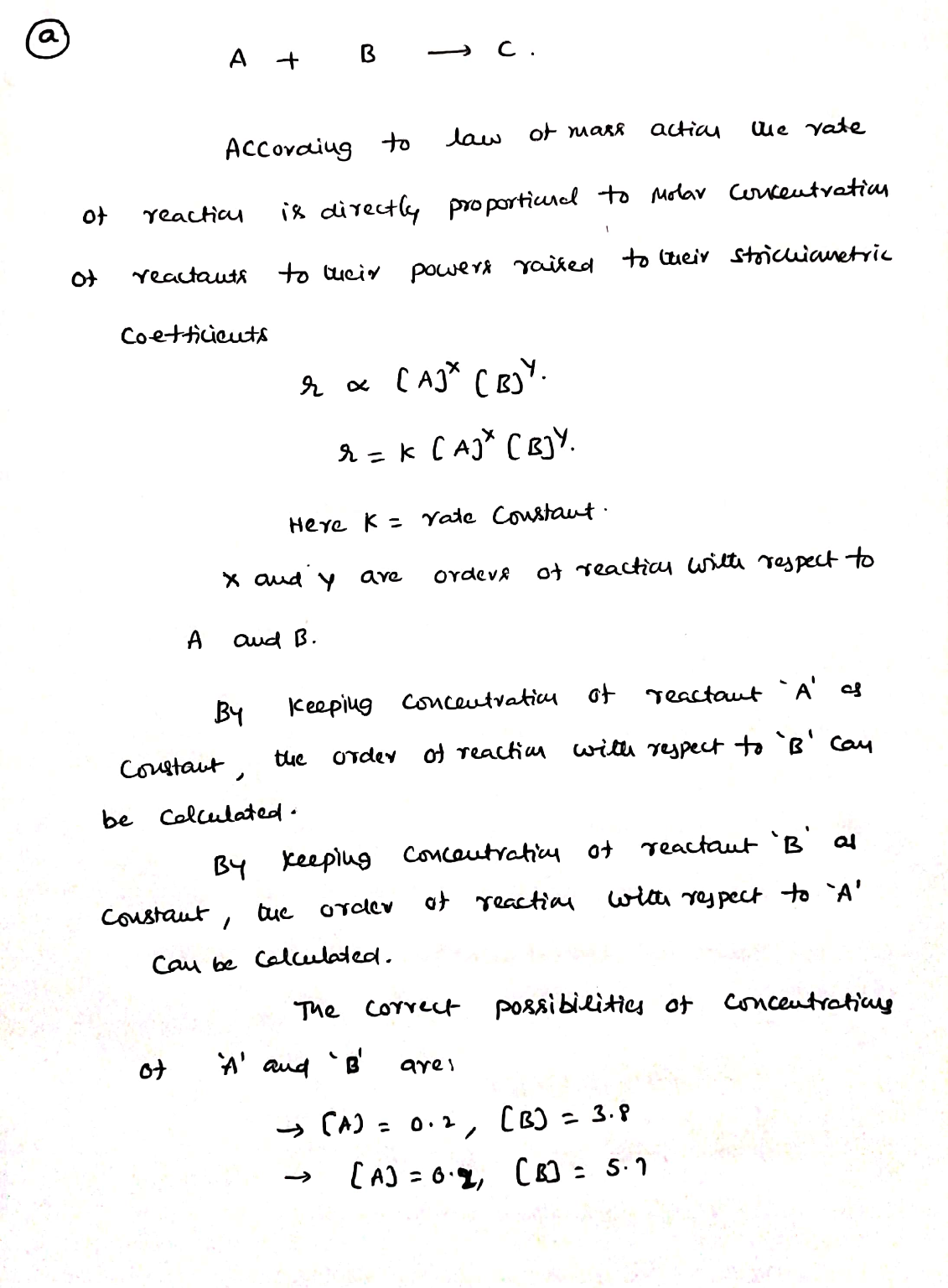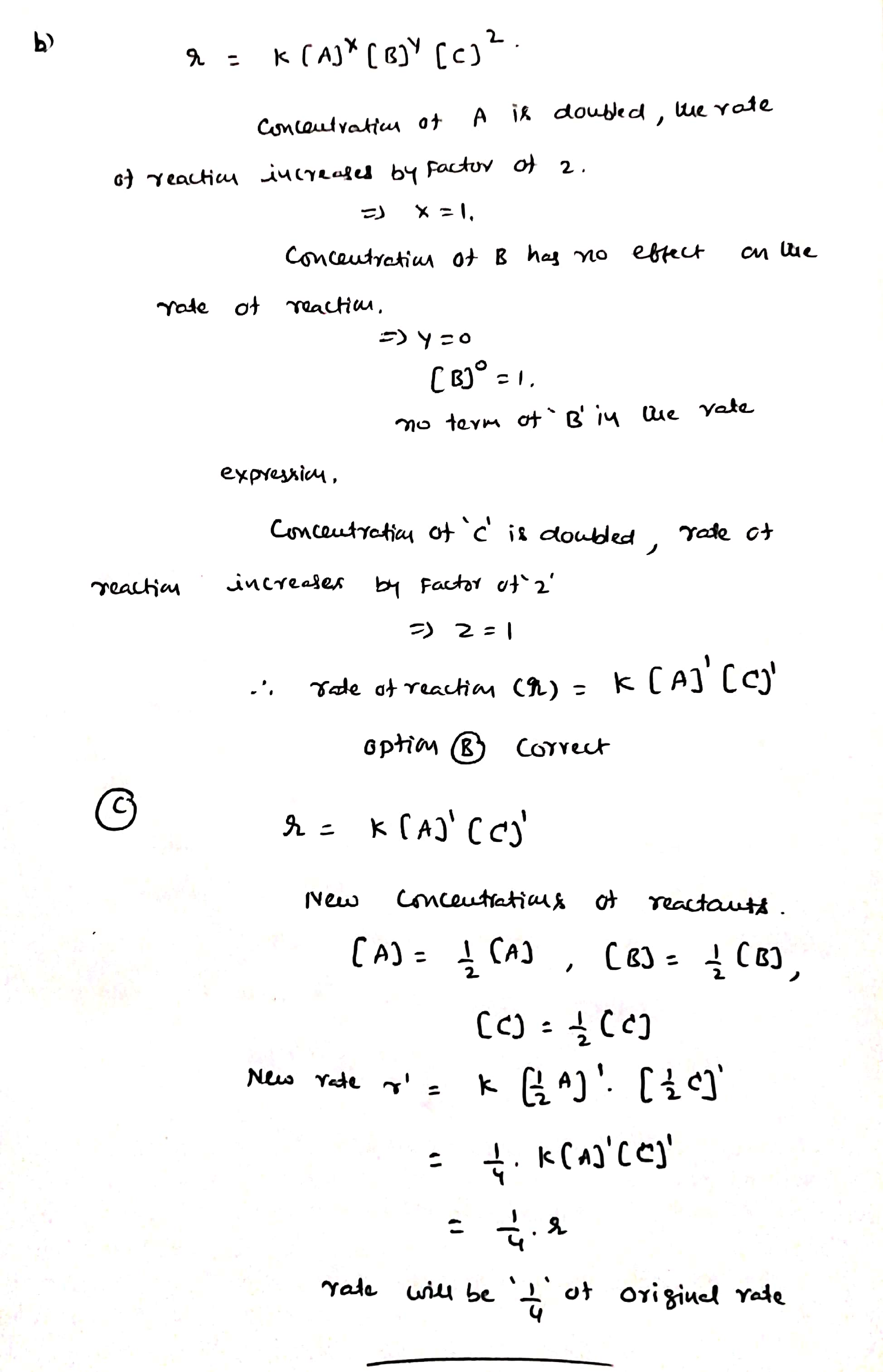#### Earn Coins

Coins can be redeemed for fabulous gifts.

Similar Homework Help Questions
• ### You and your lab partner are studying the rate of a reaction, A + B -->...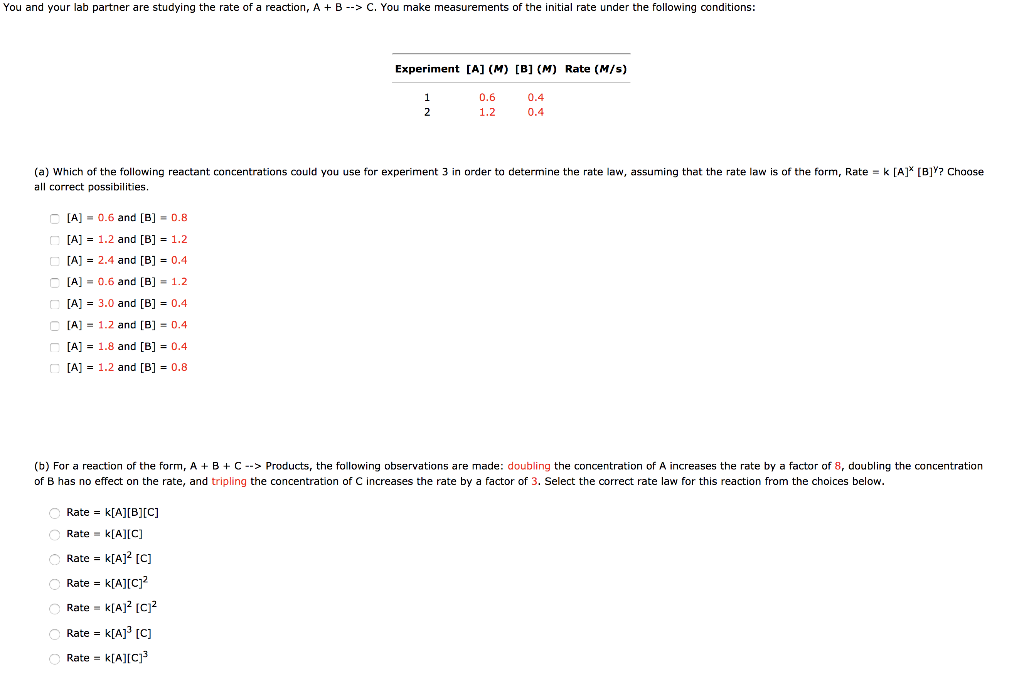You and your lab partner are studying the rate of a reaction, A + B --> C. You make measurements of the initial rate under the following conditions: Experiment [A] (M) [B] (M) Rate (M/s) 0.6 0.4 (a) Which of the following reactant concentrations could you use for experiment 3 in order to determine the rate law, assuming that the rate law is of the form, Rate = k [A] [B]Y? Choose all correct possibilities. [A] = 0.6 and [B]...

• ### You and your lab partner are studying the rate of a reaction, A + B -->...

You and your lab partner are studying the rate of a reaction, A + B --> C. You make measurements of the initial rate under the following conditions: Experiment [A] (M) [B] (M) Rate (M/s) 1 0.8 0.8 2 1.6 0.8 (a) Which of the following reactant concentrations could you use for experiment 3 in order to determine the rate law, assuming that the rate law is of the form, Rate = k [A]x [B]y? Choose all correct possibilities. [A]...

• ### You and your lab partner are studying the rate of a reaction, A + B -->...

You and your lab partner are studying the rate of a reaction, A + B --> C. You make measurements of the initial rate under the following conditions: Experiment [A] (M) [B] (M) Rate (M/s) 1 1.8 0.9 2 3.6 0.9 (a) Which of the following reactant concentrations could you use for experiment 3 in order to determine the rate law, assuming that the rate law is of the form, Rate = k [A]x [B]y? Choose all correct possibilities. [A]...

• ### An experiment was conducted to determine the rate law for the reaction A2(g)+B(g)→A2B(g) . The table...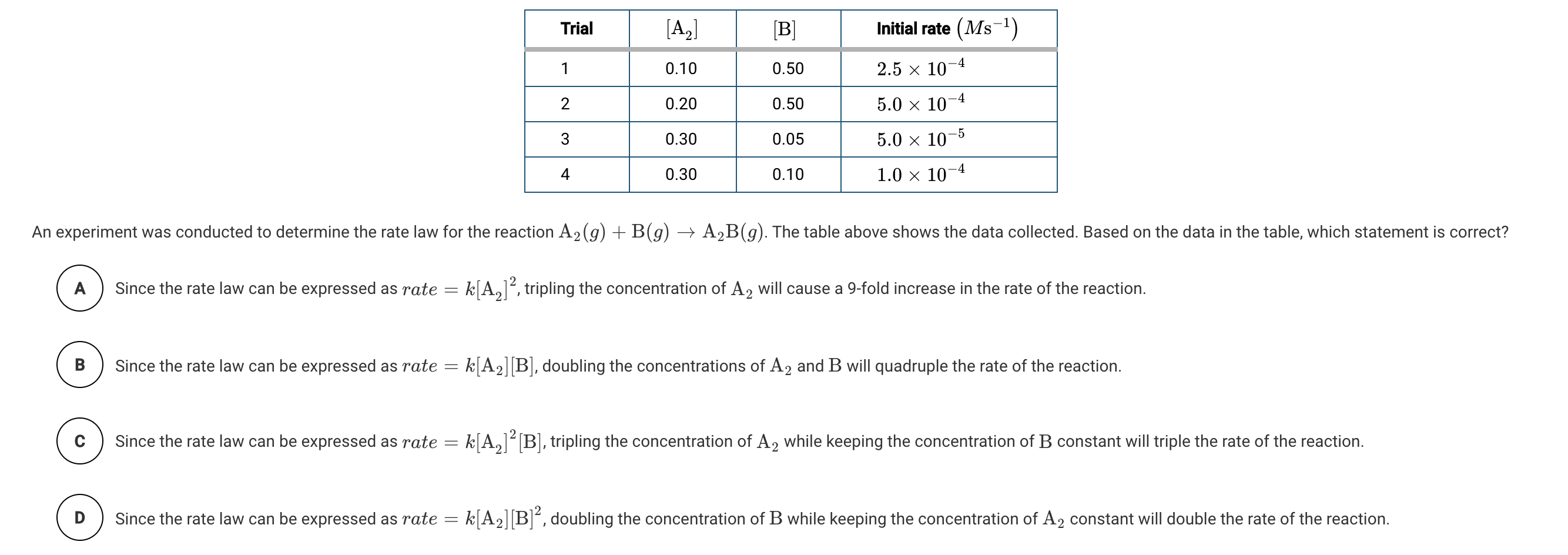An experiment was conducted to determine the rate law for the reaction A2(g)+B(g)→A2B(g) . The table above shows the data collected. Based on the data in the table, which statement is correct? Trial 1 [A2] 0.10 [B] 0.50 0.20 0.50 Nm + Initial rate (Ms-1) 2.5 10-4 5.0 x 10-4 5.0 x 10-5 1.0 x 10-4 0.30 0.05 1 0.30 0.10 An experiment was conducted to determine the rate law for the reaction A2(g) +B(g) → A2B(g). The table above...

• ### A reaction obeys the rate law: rate = k [A]2[B]. At some set of concentrations, the...

A reaction obeys the rate law: rate = k [A]2[B]. At some set of concentrations, the rate is found to be 8.2 x 10-2 M/sec. What will be the rate of reaction if the concentration of A is halved and the concentration of B is doubled? A. 4.1 x 10-2 M/sec B. 8.2 x 10-2 M/sec C. 5.0 x 10-1 M/sec D. 1.6 x 10-1 M/sec

• ### For a reaction that follows the general rate law, rate = k[A]2 [B], what will happen...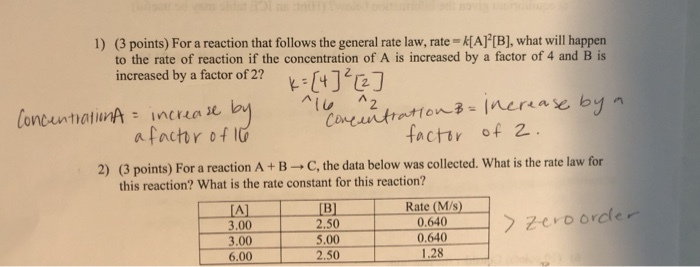For a reaction that follows the general rate law, rate = k[A]2 [B], what will happen to the rate of reaction if the concentration of A is increased by a factor of 4 and B is increased by a factor of 2? 1) (3 points) For a reaction that follows the general rate law, rate = k[A]”[B], what will happen to the rate of reaction if the concentration of A is increased by a factor of 4 and B is...

• ### 7. Consider the following reaction A+B - products. The rate law was found to be Rate...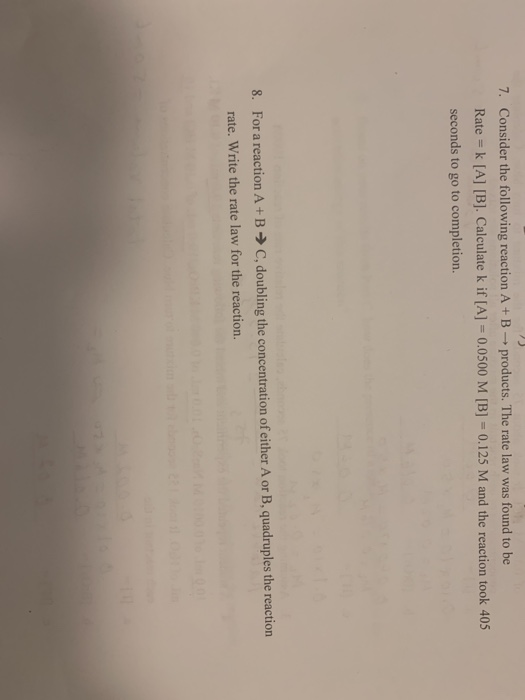7. Consider the following reaction A+B - products. The rate law was found to be Rate = k [A] [B]. Calculate k if [A] = 0.0500 M [B] = 0.125 M and the reaction took 405 seconds to go to completion. 8. For a reaction A+B → C, doubling the concentration of either A or B, quadruples the reaction rate. Write the rate law for the reaction.

• ### 2.c and 2.d 2. The rate law for the reaction A+B a. Write the rate law...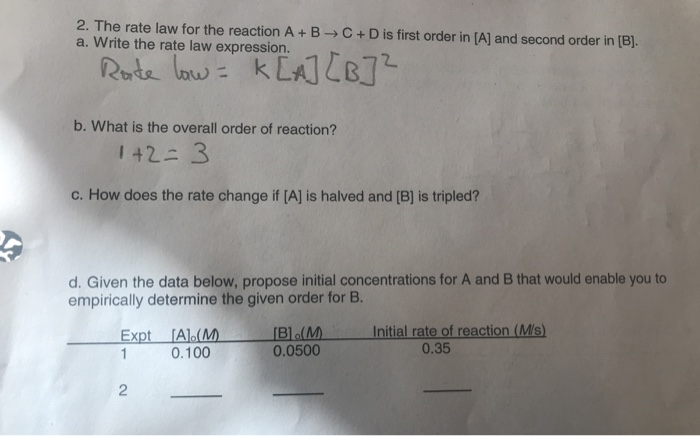2.c and 2.d 2. The rate law for the reaction A+B a. Write the rate law expression. C + D is first order in [A] and second order in [B]. Rate low- k[A] [B]² b. What is the overall order of reaction? 1 +2= 3 c. How does the rate change if [A] is halved and [B] is tripled?! d. Given the data below, propose initial concentrations for A and B that would enable you to empirically determine the given...

• ### 1. The initial reaction rate of a hypothetical reaction | A +B →G was measured at 25°C for several different starting c...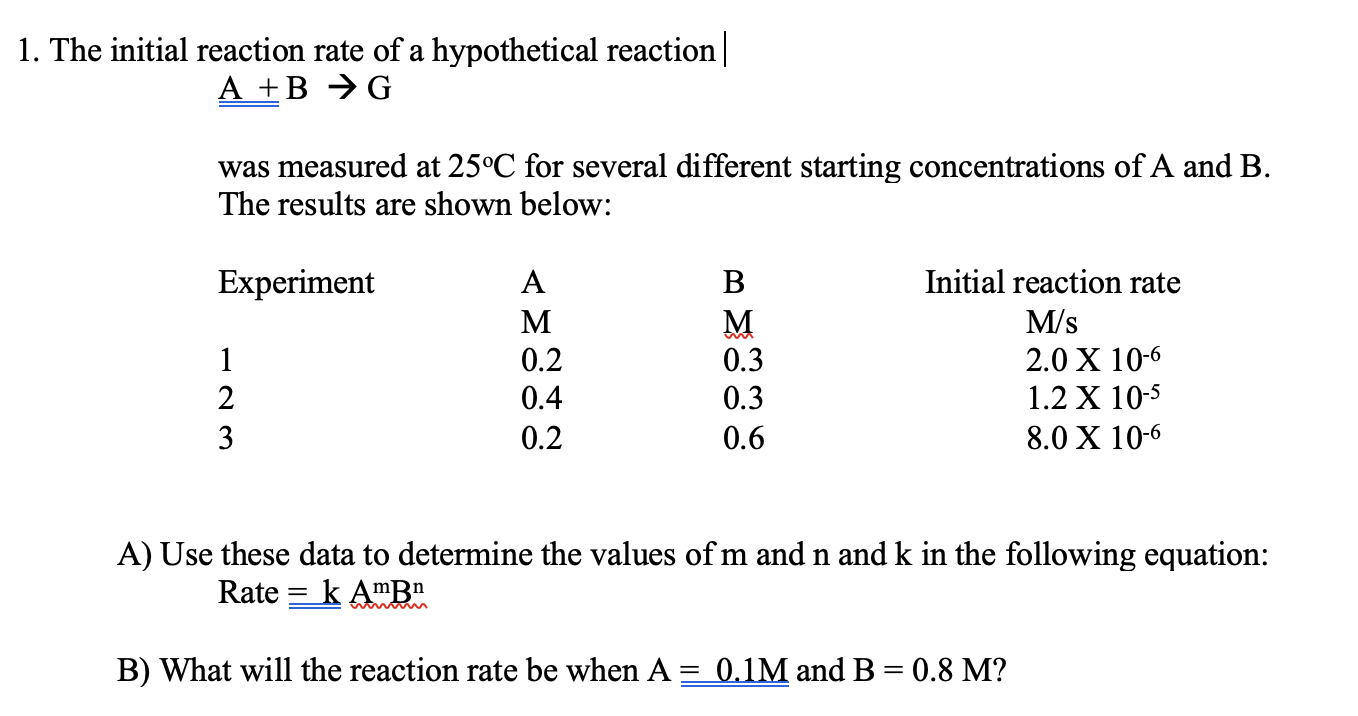1. The initial reaction rate of a hypothetical reaction | A +B →G was measured at 25°C for several different starting concentrations of A and B. The results are shown below: Experiment 0.2 Initial reaction rate M/s 2.0 X 10-6 1.2 X 10-5 8.0 X 10-6 0.4 0.3 0.6 0.2. A) Use these data to determine the values of m and n and k in the following equation: Rate = k AmBn B) What will the reaction rate be when...

• ### In a study of the reaction A + B + C → products at constant temperature,...

In a study of the reaction A + B + C → products at constant temperature, it was found that: 1. Holding [A] and [B] constant and halving the concentration of C causes the rate to proceed 2.828 times faster. 2. Holding [B] and [C] constant and doubling the concentration of A causes the reaction to proceed four times faster. 3. Doubling the concentrations of A, B, and C simultaneously, causes the reaction to proceed 1.414 times faster. The experimental...